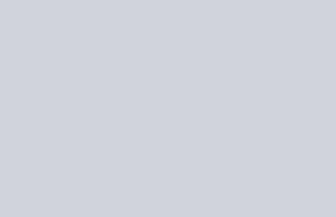The area of the region
Question:

The area of the region

$A=[(x, y): 0 \leq y \leq x|x|+1$ and $-1 \leq x \leq 1]$

in sq. units, is :

1. $\frac{2}{3}$

2. $\frac{1}{3}$

3. 2

4. $\frac{4}{3}$

Correct Option: , 3

Solution:

The graph is a follows$\int_{-1}^{0}\left(-x^{2}+1\right) d x+\int_{0}^{1}\left(x^{2}+1\right) d x=2$